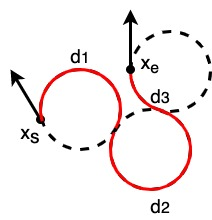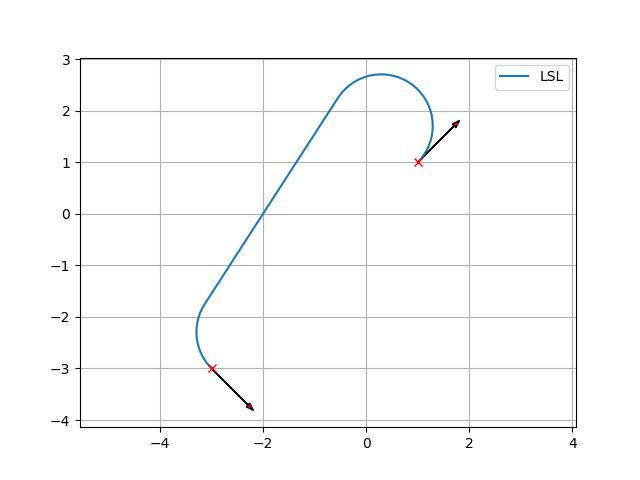# Dubins path planning

A sample code for Dubins path planning.## Dubins path

Dubins path is a analytical path planning algorithm for a simple car model.

It can generates a shortest path between two 2D poses (x, y, yaw) with maximum curvature constraint and tangent(yaw angle) constraint.

Generated paths consist of 3 segments of maximum curvature curves or a straight line segment.

Each segment type can is categorized by 3 type: ‘Right turn (R)’ , ‘Left turn (L)’, and ‘Straight (S).’

Possible path will be at least one of these six types: RSR, RSL, LSR, LSL, RLR, LRL.

Dubins path planner can output each segment type and distance of each course segment.

For example, a RSR Dubins path is:Each segment distance can be calculated by:

$$\alpha = mod(-\theta)$$

$$\beta = mod(x_{e, yaw} - \theta)$$

$$p^2 = 2 + d ^ 2 - 2\cos(\alpha-\beta) + 2d(\sin\alpha - \sin\beta)$$

$$t = atan2(\cos\beta - \cos\alpha, d + \sin\alpha - \sin\beta)$$

$$d_1 = mod(-\alpha + t)$$

$$d_2 = p$$

$$d_3 = mod(\beta - t)$$

where $$\theta$$ is tangent and d is distance from $$x_s$$ to $$x_e$$

A RLR Dubins path is:Each segment distance can be calculated by:

$$t = (6.0 - d^2 + 2\cos(\alpha-\beta) + 2d(\sin\alpha - \sin\beta)) / 8.0$$

$$d_2 = mod(2\pi - acos(t))$$

$$d_1 = mod(\alpha - atan2(\cos\beta - \cos\alpha, d + \sin\alpha - \sin\beta) + d_2 / 2.0)$$

$$d_3 = mod(\alpha - \beta - d_1 + d_2)$$

You can generate a path from these information and the maximum curvature information.

A path type which has minimum course length among 6 types is selected, and then a path is constructed based on the selected type and its distances.

## API

PathPlanning.DubinsPath.dubins_path_planner.plan_dubins_path(s_x, s_y, s_yaw, g_x, g_y, g_yaw, curvature, step_size=0.1, selected_types=None)[source]

Plan dubins path

Parameters
• s_x (float) – x position of the start point [m]

• s_y (float) – y position of the start point [m]

• s_yaw (float) – yaw angle of the start point [rad]

• g_x (float) – x position of the goal point [m]

• g_y (float) – y position of the end point [m]

• g_yaw (float) – yaw angle of the end point [rad]

• curvature (float) – curvature for curve [1/m]

• step_size (float (optional)) – step size between two path points [m]. Default is 0.1

• selected_types (a list of string or None) – selected path planning types. If None, all types are used for path planning, and minimum path length result is returned. You can select used path plannings types by a string list. e.g.: [“RSL”, “RSR”]

Returns

• x_list (array) – x positions of the path

• y_list (array) – y positions of the path

• yaw_list (array) – yaw angles of the path

• modes (array) – mode list of the path

• lengths (array) – arrow_length list of the path segments.

Examples

You can generate a dubins path.

>>> start_x = 1.0  # [m]
>>> start_y = 1.0  # [m]
>>> end_x = -3.0  # [m]
>>> end_y = -3.0  # [m]
>>> curvature = 1.0
>>> path_x, path_y, path_yaw, mode, _ = plan_dubins_path(
start_x, start_y, start_yaw, end_x, end_y, end_yaw, curvature)
>>> plt.plot(path_x, path_y, label="final course " + "".join(mode))
>>> plot_arrow(start_x, start_y, start_yaw)
>>> plot_arrow(end_x, end_y, end_yaw)
>>> plt.legend()
>>> plt.grid(True)
>>> plt.axis("equal")
>>> plt.show()